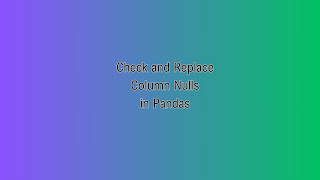### How to Check Column Nulls and Replace: PandasHere is a post that shows how to count Nulls and replace them with the value you want in the Pandas Dataframe. We have explained the process in two steps - Counting and Replacing the Null values. Count null values (column-wise) in Pandas ## count null values column-wise null_counts = df.isnull(). sum() print(null_counts) ``` Output: ``` Column1    1 Column2    1 Column3    5 dtype: int64 ``` In the above code, we first create a sample Pandas DataFrame `df` with some null values. Then, we use the `isnull()` function to create a DataFrame of the same shape as `df`, where each element is a boolean value indicating whether that element is null or not. Finally, we use the `sum()` function to count the number of null values in each column of the resulting DataFrame. The output shows the count of null values column-wise. to count null values column-wise: ``` df.isnull().sum() ``` ##Code snippet to count null values row-wise: ``` df.isnull().sum(axis=1) ``` In the above code, `df` is the Panda

# These 10 Skills You Need to Become Data Analyst

To become a data analyst with Python, there are several technical skills you need to learn. Here are the key ones:

## #1 Python Programming

Python is widely used in data analysis due to its simplicity, versatility, and the availability of powerful libraries. You should have a strong understanding of Python fundamentals, including data types, variables, loops, conditional statements, functions, and file handling.

## #2 Data Manipulation Libraries

Familiarize yourself with libraries like NumPy and Pandas, which are essential for data manipulation and analysis. NumPy provides support for efficient numerical operations, while Pandas offers data structures (e.g., DataFrames) for easy data manipulation, cleaning, and transformation.

## #3 Data Visualization

Gain proficiency in data visualization libraries like Matplotlib and Seaborn. These libraries enable you to create insightful visual representations of data, such as line plots, scatter plots, bar charts, histograms, and heatmaps.

## #4 SQL (Structured Query Language)

SQL is crucial for interacting with databases, which are often used to store and retrieve large datasets. Understanding SQL allows you to extract data, perform aggregations, filter records, and join tables. You can use Python libraries like SQLAlchemy to connect to databases and execute SQL queries.

## #5 Statistics and Probability

A solid foundation in statistics and probability theory is essential for data analysis. Topics like hypothesis testing, regression analysis, probability distributions, and sampling methods will help you make informed decisions and draw meaningful insights from data.

## #6 Exploratory Data Analysis (EDA)

EDA involves examining and summarizing data to identify patterns, anomalies, and relationships. You should learn techniques such as summary statistics, data profiling, data cleaning, and handling missing values. Python libraries like Pandas and NumPy are commonly used in EDA.

## #7 Machine Learning

Although not strictly required for data analysis, knowledge of machine learning techniques is highly beneficial. Understanding concepts like supervised and unsupervised learning, feature engineering, model evaluation, and cross-validation can enhance your data analysis capabilities. Python offers popular machine learning libraries such as Scikit-learn and TensorFlow.

## #8 Data Wrangling and Cleaning

Real-world data is often messy and requires cleaning and preprocessing before analysis. Learn techniques to handle missing data, outliers, and inconsistencies in datasets. Python libraries like Pandas provide functions for data cleaning and transformation.

## #9 Data Analysis Libraries

Explore additional Python libraries like SciPy (scientific computing), StatsModels (statistical modeling), and sci-kit-learn (machine learning). These libraries offer a wide range of tools and functions that can support advanced data analysis tasks.

## #10 Communication and Documentation

While not strictly technical skills, effective communication, and documentation are crucial for data analysts. You should be able to present your findings and insights clearly to both technical and non-technical audiences. Learn how to create visually appealing charts and graphs, write clear reports, and effectively communicate your analysis.

The bottom line

Remember that becoming a proficient data analyst is a continuous learning process. Stay updated with the latest trends, explore new libraries, participate in online communities, and work on real-world projects to further enhance your skills.